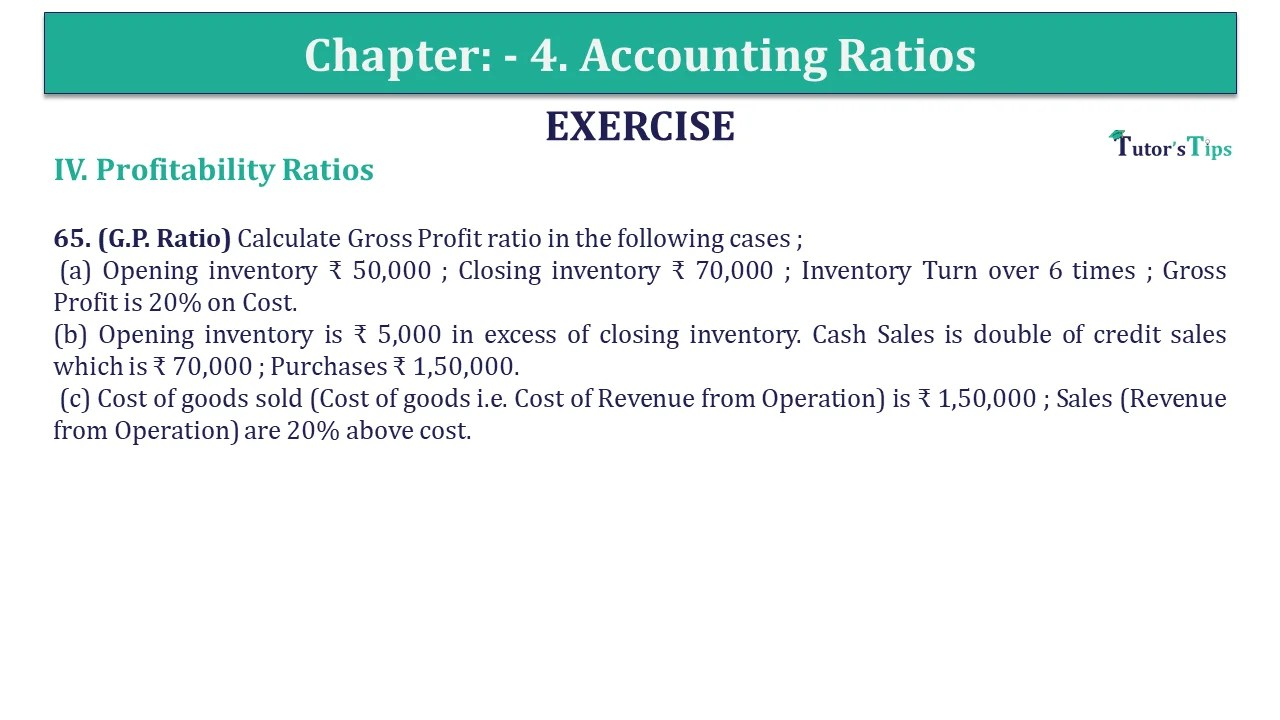# Question 65 Chapter 4 of +2-B – USHA Publication 12 ClassQ-65- CH-4 Book 2 - Usha Pub. +2 Book 2020 - Solution

Question 65 Chapter 4 of +2-B

Profitability Ratio

65. (G.P. Ratio) Calculate Gross Profit ratio in the following cases ;
(a) Opening inventory ₹ 50,000 ; Closing inventory ₹ 70,000 ; Inventory Turn over 6 times ; Gross Profit is 20% on Cost.
(b) Opening inventory is ₹ 5,000 in excess of closing inventory. Cash Sales is double of credit sales which is ₹ 70,000 ; Purchases ₹ 1,50,000.
(c) Cost of goods sold (Cost of goods i.e. Cost of Revenue from Operation) is ₹ 1,50,000 ; Sales (Revenue from Operation) are 20% above cost.

## The solution of Question 65 Chapter 4 of +2-B: –

 (a) Inventory Turnover Ratio = Cost of Goods Sold Average Inventory Average Inventory = Opening inventory +Closing inventory 2 = ₹ 50,000 + ₹ 70,000 2 = ₹ 60,000 6 = Cost of Goods Sold ₹ 60,000 Cost of Goods Sold = ₹ 60,000 x 6 = ₹ 3,60,000 Gross Profit is 20% on Cost Gross Profit = 20% of Cost of goods sold
 = 20 X ₹ 3,60,000 100 = ₹ 72,000 Sales = Cost of goods sold + Gross Profit = ₹ 3,60,000 + ₹ 72,000 = ₹ 4,32,000 Gross Profit Ratio = Gross Profit X 100 Sales = ₹ 72,000 X 100 ₹ 4,32,000 = 16.67%

 (b) Credit Sales = ₹ 8,40,000 X 100 ₹ 10,00,000 = 84%
 (b) Credit Sales = ₹ 70,000 = ₹ 70,000 x 2 = ₹ 1,40,000 Total Sales = ₹ 1,40,000 + ₹ 70,000 = ₹ 2,10,000 Purchase = ₹ 1,50,000 Opening Inventory = Closing Inventory + ₹ 5,000 Gross Profit = Sales – Purchases – Opening Inventory + Closing Inventory = ₹ 2,10,000 – ₹ 1,40,000 – ₹ 5,000 = ₹ 55,000
 Gross Profit Ratio = ₹ 55,000 X 100 ₹ 2,10,000 = 26.19%
 (c) Cost of goods sold = ₹ 1,50,000 Sales 20% above cost = ₹ 1,50,000 + 20%
 = 20 X ₹ 1,50,000 100 = ₹ 30,000 Sales = ₹ 1,50,000 + ₹ 30,000 = ₹ 1,80,000 Gross Profit = ₹ 1,80,000 + ₹ 1,50,000 = ₹ 30,000
 Gross Profit Ratio = ₹ 30,000 X 100 ₹ 1,80,000 = 16.67%

Also, Check out the solved question of previous Chapters: –